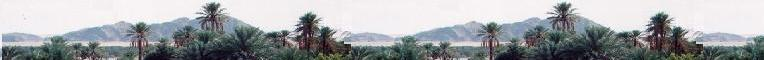# Mathématiques 2: Optimization

### 1. Definitions


Optimization of a situation refers to
maximize or minimize that situation. We often
want to maximize profit and revenue and minimize
time and losses.

We want to optimize some simple situations using graphs.
Complex optimization problems, to be solved, recquire
the use of derivatives and operational research.

Using the graphs, the steps to solve an optimization problem are:

3 - Understand the word-problem,
4 - Draw a picture or graph if appropriate,
5 - Identify the constants, the variables, and what is to be optimized,
6 - Set the constraints,
7 - Plot the graphs of the constraints,
8 - Draw a polygon of constraints in the whole graph,
9 - Identify the objective function (max. or min.),
10 - Evaluate the objective function at the polygon's vertices.
11 - Make a decision (written response).

All the acceptable solutions can be found inside the
polygon of constraints.

The objective function determine the best solution. Depending
on the situation, this best solution gives the highest value
(maximum) or lowest value ( minimum).



### 2.Three cases of situation

Generally, we have three cases:

Case 1:

The optimal solution is unique and situated
at a vertex of the polygon of constraints.

Case 2:

If the line of the objective function is parallel to
a segment of the polygon, the optimal value in located
between the two consecutive vertices that form this
segment.

Case 3:

If we cannot build a polygon with the given
constaraints, we do not have solutions.Web ScientificSentence

 chimie labs | scientific sentence | java | php | green cat | contact |